Warning

This documents an unmaintained version of NetworkX. Please upgrade to a maintained version and see the current NetworkX documentation.

# fast_gnp_random_graph¶

fast_gnp_random_graph(n, p, seed=None, directed=False)[source]

Returns a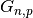random graph, also known as an Erdős-Rényi graph or a binomial graph.

Parameters: n (int) – The number of nodes. p (float) – Probability for edge creation. seed (int, optional) – Seed for random number generator (default=None). directed (bool, optional (default=False)) – If True, this function returns a directed graph.

Notes

Thegraph algorithm chooses each of the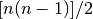(undirected) or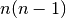(directed) possible edges with probability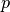.

This algorithm runs in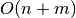time, where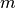is the expected number of edges, which equals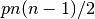. This should be faster than gnp_random_graph() whenis small and the expected number of edges is small (that is, the graph is sparse).

References

  Vladimir Batagelj and Ulrik Brandes, “Efficient generation of large random networks”, Phys. Rev. E, 71, 036113, 2005.